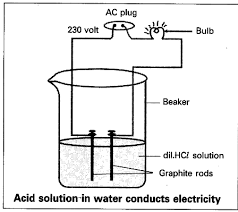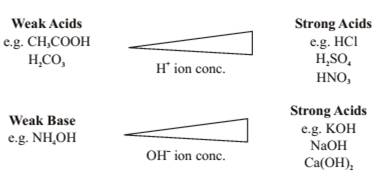### Acids Bases and Salts - Revision Notes

CBSE Class 10 Science

Revision Notes
CHAPTER – 2
ACIDS, BASES AND SALTS

 Acids Bases Sour in tasteDerived from Greek word' ACIDUS' Bitter in taste Changes blue litmus into red Changes red litmus into blue e.g. Hydrochloric acid HCl e.g. Sodium hydroxide NaOH Sulphuric acid H2SO4${H}_{2}S{O}_{4}$ Potassium hydroxide KOH Nitric acidHNO3$HN{O}_{3}$ Calcium hydroxideCa(OH)2${C}_{a}\left(OH{\right)}_{2}$ Acetic acidCH3COOH$C{H}_{3}\phantom{\rule{thickmathspace}{0ex}}COOH$ Ammonium hydroxide
• Some naturally occurring acids
 Vinegar Acetic Acid Orange Citric Acid Lemon Citric Acid Tamarind Tartaric Acid Tomato Oxalic Acid Sour milk (Curd) Lactic Acid Ant and Nettle sting Methanoic Ac
• Acid – Base Indicator:Substances which indicate the presence of an acid or base in a solution.
• Litmus solution – It is a natural indicator. It is a purple dye extracted from Lichens. Other examples are Red Cabbage and coloured petals of Petunia and turmeric.
• Olfactory indicators : Show odour changes in acidic or basic media. E.g. onion and clove .
• Acid – Base Indicators
 S.No. Name of the Indicator Colour Change With Acid Colour Change with Base A. Blue litmus solution To red No change B. Red litmus solution No change To blue C. Turmeric No change To red D. Methyl orange To red To yellow E. Phenolphthalein (colourless) No change To pink
• Dilute Acid : A dilute acid contains a small amount of acid (lower concentration of hydronium ions)and a large amount of water.
• Concentrated Acid :A concentrated acid contains a large amount of acid (higher concentration of hydronium ions)and a small amount of water.
• Chemical Properties of Acids and Bases
1. Reaction with metal

$Acid\phantom{\rule{thickmathspace}{0ex}}+\phantom{\rule{thickmathspace}{0ex}}Metal\phantom{\rule{thickmathspace}{0ex}}\to \phantom{\rule{thickmathspace}{0ex}}Salt\phantom{\rule{thickmathspace}{0ex}}+\phantom{\rule{thickmathspace}{0ex}}Hydrogen$

$2HCl+Zn\stackrel{}{\to }\phantom{\rule{thickmathspace}{0ex}}ZnC{l}_{2}\phantom{\rule{thickmathspace}{0ex}}+\phantom{\rule{thickmathspace}{0ex}}{H}_{2}$

$2HN{O}_{3}+\phantom{\rule{thickmathspace}{0ex}}Zn\stackrel{\phantom{\rule{thinmathspace}{0ex}}\phantom{\rule{thinmathspace}{0ex}}\phantom{\rule{thinmathspace}{0ex}}\phantom{\rule{thinmathspace}{0ex}}}{\to }Zn\left(N{O}_{3}{\right)}_{2}+{H}_{2}$

${H}_{2}S{O}_{4}+Zn\stackrel{}{\to }ZnS{O}_{4}+{H}_{2}$

$2C{H}_{3}COOH+Zn\stackrel{}{\to }\phantom{\rule{0ex}{0ex}}\left(C{H}_{3}COO{\right)}_{2}Zn+{H}_{2}$

• Pop test : When a burning candle is brought near a test tube containing hydrogen gas it burns with a ‘Pop’ sound. This test is conducted for examining the presence of hydrogen gas.
• Base + Metal $\stackrel{}{\to }$ Salt + Hydrogen

$NaOH+Zn\phantom{\rule{thickmathspace}{0ex}}\stackrel{}{\to }\phantom{\rule{thickmathspace}{0ex}}\underset{Sodium\phantom{\rule{thickmathspace}{0ex}}Zincate}{N{a}_{2}Zn{O}_{2}+{H}_{2}}$

Note– Such reactions are not possible with all the metals.

• Actions of Acids with metal Carbonates and metal bicarbonates

• Lime water Test : On passing the evolved $C{O}_{2}$ gas through lime water, we find that lime water turns milky.

• $C{O}_{2}$ the following reaction takes place

$CaC{O}_{3\left(s\right)}+{H}_{2}{O}_{\left(l\right)}+C{O}_{2\left(g\right)}\stackrel{}{\to }\phantom{\rule{0ex}{0ex}}\underset{\text{(soluble}\phantom{\rule{thinmathspace}{0ex}}\phantom{\rule{thickmathspace}{0ex}}\text{in water)}}{Ca{\left(HC{O}_{3}\right)}_{2}aq}$

• Reaction of acids and bases with each other to give salt and water are called Neutralisation Reactions

$Base\phantom{\rule{thickmathspace}{0ex}}+\phantom{\rule{thickmathspace}{0ex}}Acid\phantom{\rule{thickmathspace}{0ex}}\stackrel{}{\to }\phantom{\rule{thickmathspace}{0ex}}Salt\phantom{\rule{thickmathspace}{0ex}}+\phantom{\rule{thickmathspace}{0ex}}Water$

e.g.

• Reactions of metal oxides with acids

$MetalOxide+Acid\stackrel{}{\to }Salt+Water$

$\underset{Copperoxide}{CuO}+\underset{Hydrochloric\phantom{\rule{thinmathspace}{0ex}}\phantom{\rule{thinmathspace}{0ex}}acid}{HCl}\to \phantom{\rule{0ex}{0ex}}\underset{Copper\phantom{\rule{thinmathspace}{0ex}}\phantom{\rule{thinmathspace}{0ex}}Chloride}{CuC{l}_{2}}+\underset{Water}{{H}_{2}O}$

Note : Appearance of blue green colour of the solution because of formation of $CuC{l}_{2}$.

Metallic oxides are said to be basic in nature because they give salt and water on reacting with acids.Some metallic oxides react with both acids and base and are called AMPHOTER-
IC OXIDES.

• Reaction of Non Metallic Oxide with Base

Non-metallic oxide+ Base$\stackrel{}{\to }$ Salt + Water

$C{O}_{2}+Ca\left(OH{\right)}_{2}\stackrel{}{\to }CaC{O}_{3}+{H}_{2}O$

Note : Non Metallic oxides are said to be acidic in nature because on reacting with a base they produce salt and water.

• All acidic solutions conduct electricity because of formation of $\left({H}^{+}\right)$ions in aq. solution.• Glowing of bulb indicates that there is a flow of electric current through the solution.
• Acids or bases in a Water Solution

Acids produce${H}^{+}$ions in the presence of water

$HCl\phantom{\rule{thickmathspace}{0ex}}+\phantom{\rule{thickmathspace}{0ex}}\phantom{\rule{thickmathspace}{0ex}}{H}_{2}O\phantom{\rule{thickmathspace}{0ex}}\stackrel{\phantom{\rule{thickmathspace}{0ex}}}{\to }\phantom{\rule{thickmathspace}{0ex}}{H}_{3}{O}^{+}+C{l}^{-}$

${H}_{3}{O}^{+}\phantom{\rule{thickmathspace}{0ex}}\phantom{\rule{thickmathspace}{0ex}}$ : Hydronium ion.

${H}^{+}$ion cannot exist alone. It exists as ${H}^{+}\left(aq\right)\phantom{\rule{thickmathspace}{0ex}}or\phantom{\rule{thickmathspace}{0ex}}\left({H}_{3}{O}^{+}\right)$ hydronium ion.

i.e. Base provide $O{{H}^{-}}_{\left(aq\right)}$ ions in the presence of water

$NaO{H}_{\left(s\right)}\stackrel{{H}_{2}O}{\to }N{{a}^{+}}_{\left(aq\right)}+O{{H}^{-}}_{\left(aq\right)}$

$KO{H}_{\left(s\right)}\stackrel{{H}_{2}O}{\to }{{K}^{+}}_{\left(aq\right)}+O{{H}^{-}}_{\left(aq\right)}$

$Mg\left(OH{\right)}_{{2}_{\left(s\right)}}\stackrel{{H}_{2}O}{\to }M{g}^{2+}\left(aq\right)+2O{H}^{-}\left(aq\right)$

• Alkalis

All bases do not dissolve in water. An alkali is a base that dissolves in water.Common alkalis are

• NaOH Sodium hydroxide
• KOH Potassium hydroxide
• Calcium hydroxide
• Ammonium hydroxide

Note : All alkalis are bases but all bases are not alkalis.

• Precaution must be taken while mixing acid or base with water. The acid must always be added to water with constant stirring as it is a highly exothermic reaction.

When an acid or a base is mixed with water they become dilute. This results in the decrease in the concentration of per unit volume in acids and bases respectively,i.e. no. of ${H}^{+}ion$and $O{H}^{-}ion$ reduces.

• Strength of an Acid or Base

Strength of acids and bases depends on the no. of ${H}^{+}ion$and $O{H}^{-}ion$produced respectively.

With the help of a universal indicator we can find the strength of an acid or base as it shows different colours at different concentrations of hydrogen ions in a solution.

A scale for measuring hydrogen ion conc. in a solution called pH scale has been developed.

pH= Potenz in German means power.

This scale measures from 0 (very acidic) to 14 (very alkaline) 7 indicates

Neutral pH (water is neutral).

pH paper : Is a paper which is used for measuring pH

Variation of PH

S. No. PH Colour of the Nature of Solution ${H}^{+}ion$ Conc. $O{H}^{-}ion$ Conc.

Value pH Paper

 1 0 Dark red Highly acidic Very high Very low 2 4 Orange or yellow Acidic High Low 3 7 Green Neutral Equal Equal 4 10 Bluish green or blue Alkaline Low High 5 14 Dark blue or violet highly basic very low Very highImportance of pH in our daily life

• Importance of pH in our digestive system – Our stomach produces hydrochloric acid. This dilute hydrochloric acid help in digestion of food. In case of indigestion our stomach produces acid in a very large quantity because of which we feel pain and irritation in our stomach (ACIDITY).To get relief from this pain ,antacids are used. These antacids neutralize the excess acid because they are basic in nature and we get relief.
• pH of Acid Rain : When pH of rain water is less than 5.6 it is called acid rain. Flow of acidic rain in water bodies makes them acidic causing a threat to the survival of aquatic life.It also results in damage of structures made with marble like Taj Mahal.
• pH of Soil : Plants require a specific range of pH for their healthy growth. If pH of soil of any particular place is less or more than normal then the farmers add suitable chemicals to it. The addition of these chemicals of presences of excessive damage the nutrients of the soil and decrease its natural fertility.
• Our body functions between the pH range of 7.0 to 7.8. Living organisms can survive only in the narrow range of pH change.
• Tooth decay and pH : Bacteria present in the mouth produces acids by degradation of sugar and food particles remaining in the mouth. Tooth decay begins below the pH 5.6. Using toothpaste which is generally basic, can neutralise the excess acid and prevent tooth decay.
• Bee sting or Nettle sting contains methanoic acid which causes pain and irritation. Using a weak base like baking soda neutralises the acid giving relief.

Salts and their Derivation

 S. No. Name of Salt Formula Derived from Derived from 1. Potassium Sulphate